### AMA Code

##### Problem Statement

Characterize the dynamics of xt where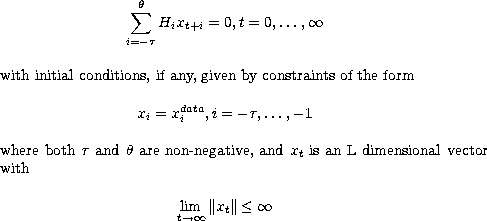##### Anderson-Moore Algorithm Output Matrices

Q - Asymptotic Linear Constraints Matrix such that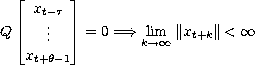for all xt satisfying the linear homogeneous system.

B - Autoregressive Representation Matrix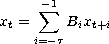satisfies the linear homogeneous system and lim k||xt+k|| <.

S - Observable Structure Matrix
determine the existence and uniqueness of an observable structure matrix, S such that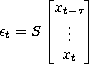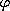,- Stochastic Transition Matrices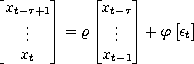The SPSolve routine computes cof(for H), cofb(for B), scof(for S), dTrans(for), and bTrans(for).